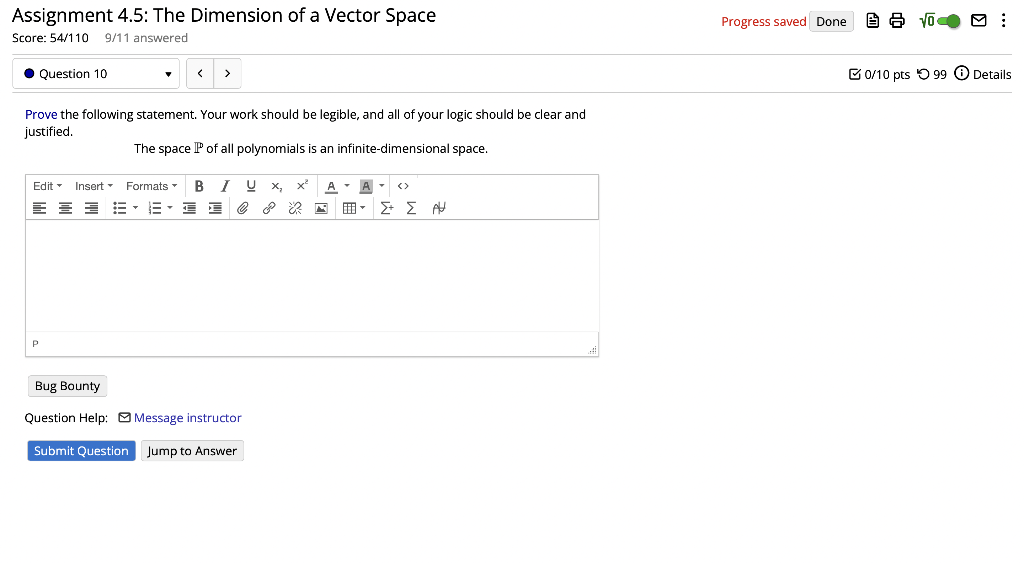# Question Assignment 4.5: The Dimension of a Vector Space Score: $$54 / 110 \quad 9 / 11$$ answered Prove the following statement. Your work should be legible, and all of your logic should be clear and justified. The space $$\mathbb{P}$$ of all polynomials is an infinite-dimensional space. Question Help: $$\square$$ Message instructorTranscribed Image Text: Assignment 4.5: The Dimension of a Vector Space Score: $$54 / 110 \quad 9 / 11$$ answered Prove the following statement. Your work should be legible, and all of your logic should be clear and justified. The space $$\mathbb{P}$$ of all polynomials is an infinite-dimensional space. Question Help: $$\square$$ Message instructor
Transcribed Image Text: Assignment 4.5: The Dimension of a Vector Space Score: $$54 / 110 \quad 9 / 11$$ answered Prove the following statement. Your work should be legible, and all of your logic should be clear and justified. The space $$\mathbb{P}$$ of all polynomials is an infinite-dimensional space. Question Help: $$\square$$ Message instructor&#12304;General guidance&#12305;The answer provided below has been developed in a clear step by step manner.Step1/2Let $$\mathrm{{\mathbf{{P}}}}$$be the space of all polynomials.Now we may prove why $$\mathrm{{\mathbf{{P}}}}$$ is an infinite denominational vector space. Suppose the dimension of $$\mathrm{{\mathbf{{P}}}}$$ is finite say n. The linear independent contains the set of more than n vectors.Consider the set $$\mathrm{{S}={\left\lbrace{1},{x},{x}^{{2}},{x}^{{3}},---,{x}^{{n}}\right\rbrace}}$$Let $$\mathrm{{a}_{{0.1}}+{a}_{{1}}.{x}+{a}_{{2}}.{x}^{{2}}+\ldots+{a}_{{n}}.{x}^{{n}}={0}}$$Then$$\mathrm{{x}={0}}$$ implies $$\mathrm{{a}_{{0}}={0}}$$Therefore $$\mathrm{{a}_{{1}}.{x}+{a}_{{2}}.{x}^{{2}}+\ldots+{a}_{{n}}.{x}^{{n}}={0}}$$Then \( \mathrm{{a}_{{1}}+{a}_{{2}}.{x}+{a}_{{3}}.{x}^{{2}}+\ldots+{a}_{{n}}.{x}^{{{n}-{1}} ... See the full answer